# Python教程-Python (x,y) 软件Python (x,y) 是一款用于工程和科学开发的免费软件。Python (x,y) 软件用于数据分析、数据可视化和数值计算。Python (x,y) 基于 Spyder 交互式科学开发环境、Python 编程语言和基于 Qt 的图形用户界面（GUI）。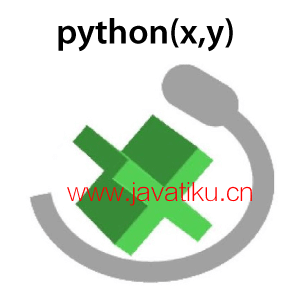## Python (x,y) 能做什么？

• 我们可以使用 Python (x,y) 软件执行简单的函数式编程任务（编程语言具有类似 MATLAB 的语法），也可以执行强大的面向对象编程任务。
• 在使用 Python (x,y) 时，我们甚至可以使用并行 Python 进行集群计算。
• 从最简单的 Python 脚本到最复杂的应用程序，我们都可以使用 Python (x,y) 软件进行科学项目开发。
• 由于其基于 Qt 的图形用户界面和 Spyder 科学开发环境，我们可以轻松地进行科学项目开发。
• 使用 Python (x,y) 软件，我们可以进行各种交互式计算，包括符号数学和 2D（二维）以及 3D 图形绘制。
• 我们甚至可以在多处理器或多核计算机上使用 Python (x,y) 进行并行计算。
• 除了上述所有任务，我们还可以使用 Python (x,y) 软件执行许多其他任务...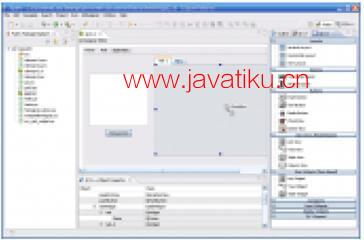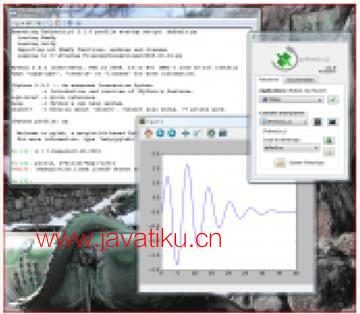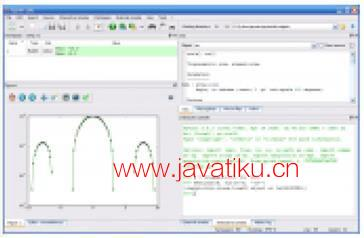## Python (x,y) 的历史

Pierre Raybaut 自 2008 年以来一直在维护、构思和开发 Python (x,y) 软件，并拥有未来与性能相关的目标（我们将在本文后面讨论）。2011 年，Gabi Davar 加入了 Python (x,y) 项目，与 Pierre Raybaut 一起成为项目的维护者。后来，2013 年，Pierre Raybaut 离开了这个项目，转而致力于其他各种项目。自 2013 年以来，Gabi Davar 一直是 Python (x,y) 项目的主要维护者。

## Python (x,y) 到底是什么

Python (x,y) 软件的原始和主要目的是帮助所有使用编译语言或解释语言的科学程序员和项目开发者转向 Python。Python (x,y) 是基于 Spyder 和 Qt 界面的科学开发导向 Python 分发软件。在 Python (x,y) 中，所有使用编译编程语言的程序员也喜欢将他们的代码“原样”包装在软件中，以便我们可以在 Python 脚本中直接调用它们。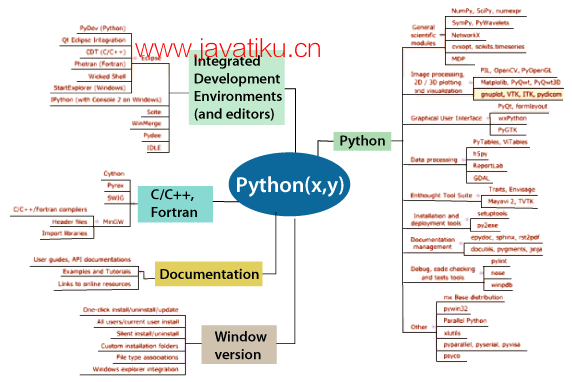• IDL 是 ITT Visual Information Solutions 的注册商标，MATLAB 是 The MathWorks 的注册商标。
• 我们可以在 Python (x,y) 软件的插件页面中直接看到 Spyder 和 Qt GUI 的界面。
• 要下载 Python (x,y) 软件并查看其插件，可以直接访问其 GitHub 上的官方网站，或使用以下链接进行下载：`https://python-xy.github.io/downloads.html`

## Python (x,y) 的主要特点

Python (x,y) 软件具有许多功能，但我们将描述一些重要的功能。

• 想要在设备上安装或卸载许多 Python 软件包的用户可以通过 Python (x,y) 软件提供的一个按钮简单地执行此操作。
• Python (x,y) 还提供了有关如何开始使用 Python、Spyder 和 Qt 界面的快速用户指南。
• Python (x,y) 可以收集开发环境工具以及面向科学的 Python 库。
• 借助 Python (x,y) 软件，我们几乎可以收集所有免费相关的文档。
• Python (x,y) 软件为我们提供了一键式安装程序，使所有用户都能轻松在其设备上安装它。

## Python (x,y) 为什么创建？

• 使用 IPython（增强的 Python shell）进行快速原型制作
• 使用 Spyder 集成开发环境提供的环境进行小型和大型项目（类似应用程序的项目）的开发。
• 用于许多科学开发目的，例如：

1. 数值模拟，
2. 图像处理物理学（实验和建模），
3. 信号处理，
4. 科学计算，
5. 科学工程开发等。

### 为什么选择 Python？

• Python 是一种开源和免费的科学语言。
• Python 易于嵌入、扩展和移植。
• Python 是一种基本面向对象的编程语言。
• 它还是一种通用语言，例如，具有丰富的输入输出（I/O）功能，拥有许多数据库库，容易在互联网上找到，具有强大的图形用户界面等等。

### 为什么选择 Qt？

PyQt 是一种非常易于使用且功能强大的库，因此我们强烈建议在 GUI（图形用户界面）开发中使用它。许多科学程序和开发，例如 2D matplotlib 图形图形等，可以轻松嵌入 PyQt 应用程序窗口中，其操作方式类似于 MATLAB。